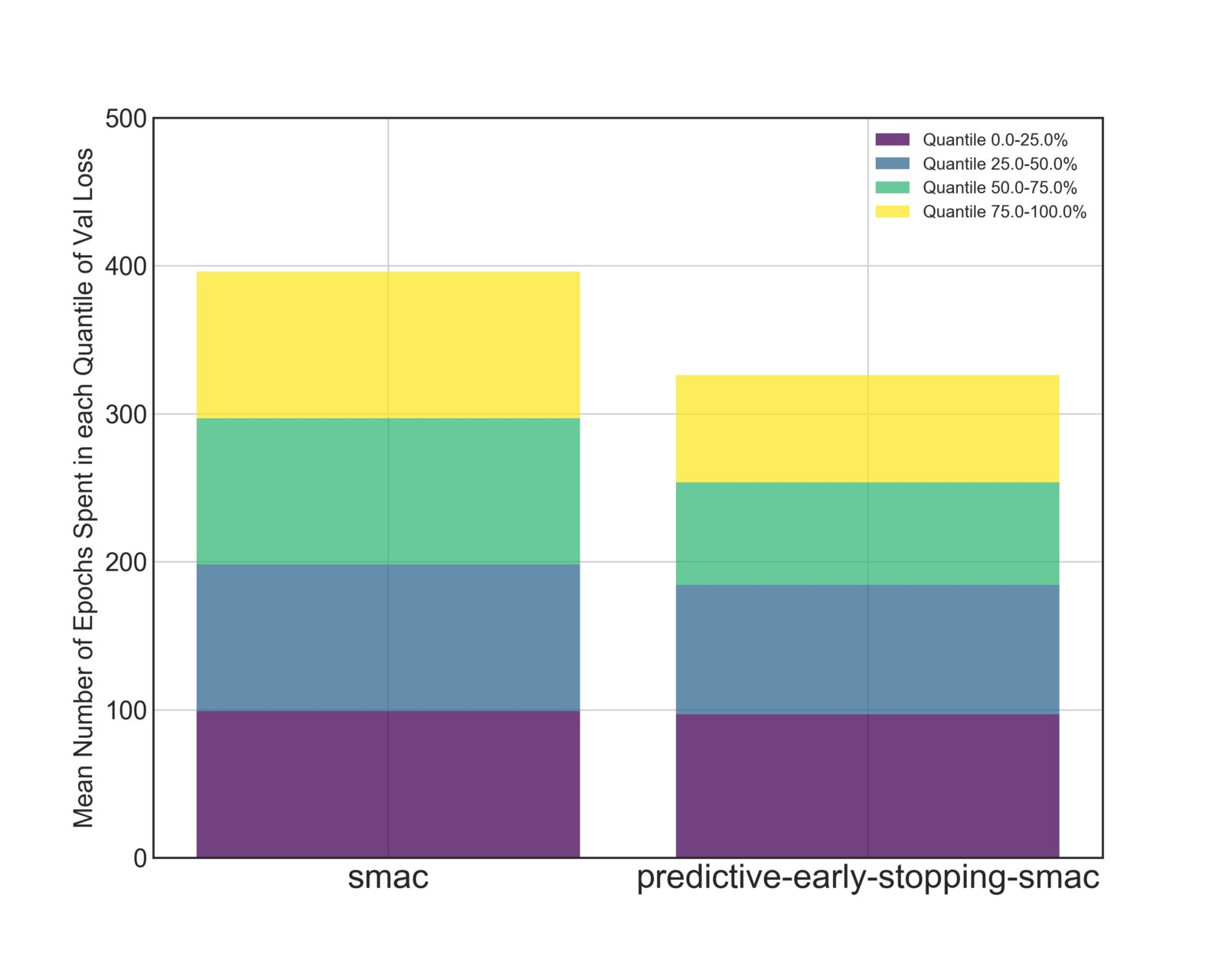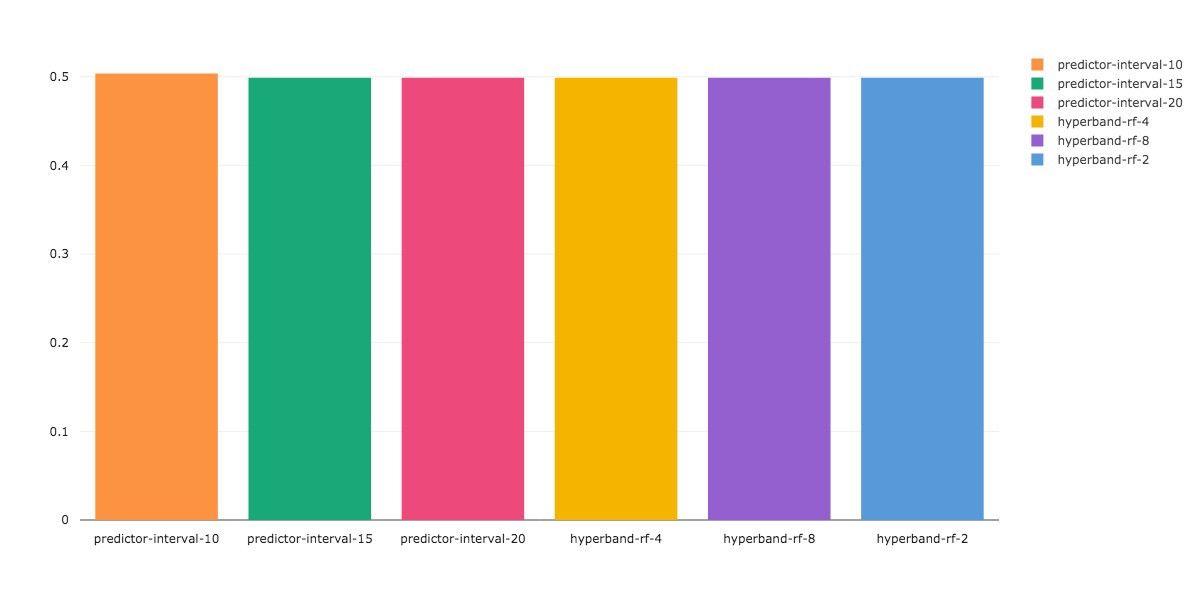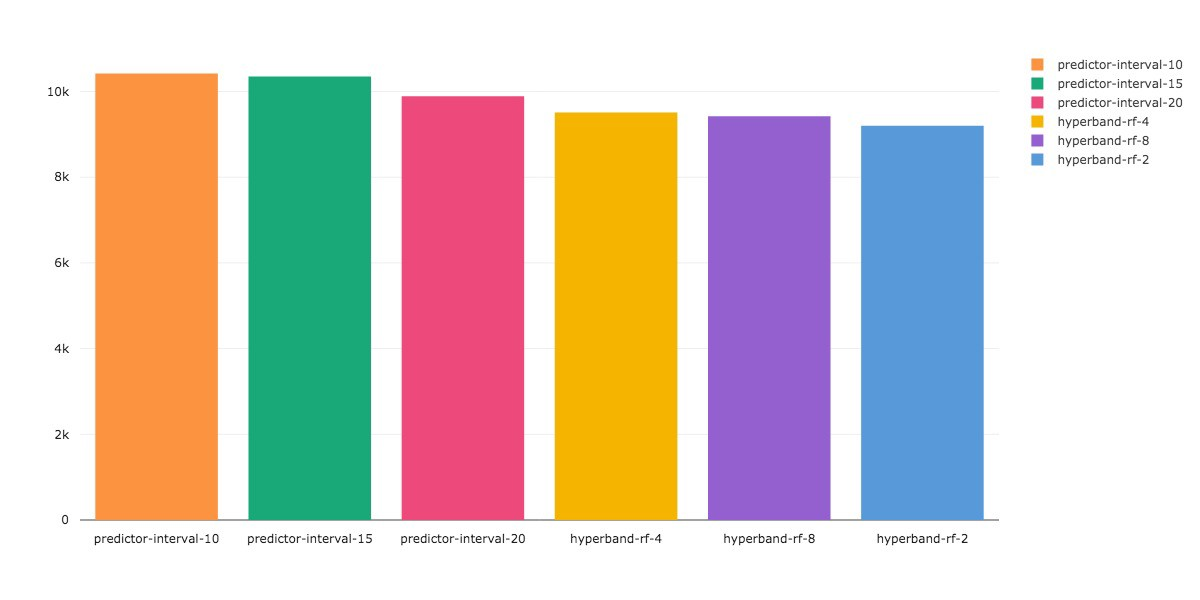Comet Launches Prompt Management Tools to revolutionize your Large Language Model workflow.

# Predictive Early Stopping – A Meta Learning Approach## Introduction

Model training is arguably the most time consuming, and computationally demanding part of the Machine Learning pipeline. Depending on the complexity of your model, or search space, it can take weeks or even months to find an adequate set of parameters that allow your model to fit the data.

Predictive Early Stopping is a state-of-the-art approach for speeding up model training and hyperparameter optimization. Our benchmarking studies have shown that Predictive Early Stopping can speed up model training by up to 30% independent of the underlying infrastructure.

We build on insights gathered from projects such as Learning Curve Extrapolation, Hyperband, and Median Stopping, in order to create a predictive model that can estimate the convergence value of a loss curve.

Comet is able to leverage model data, such as hyperparameters and loss curves, from over two million models in the public section of its platform to create a model whose predictions generalize across hyperparameters and model architectures.

In some cases we are able to provide an estimate of convergence, hundreds of epochs before it actually occurs. In addition to predicting the convergence value, our Predictive Early Stopping product provides an estimate of the probability that the current model will outperform the best model result seen in the current training sweep.

In some cases we are able to provide an estimate of convergence, hundreds of epochs before it actually occurs.

These predictions allow us to terminate the training of underperforming models, so that the search process is spent evaluating only the most promising candidates.

## Benchmarking:

We tested our Predictive Early Stopping method in three different settings:

1. A hyperparameter search that optimizes the parameters of a function that acts as a surrogate for a neural network
2. A hyperparameter search to optimize a 6 layer CNN on CIFAR10 using the SMAC optimizer, with and without predictive early stopping.
3. A hyperparameter search to optimize the same 6 layer CNN using Random Search with Hyperband vs Random Search with Predictive Early Stopping.

### Results for the Surrogate Function:

In our first test, we setup an exponentially decaying function as our surrogate for a Neural Network. We ran this surrogate model for 20 steps and determined the optimal values for the parameters of this function using Comet’s Bayesian Optimizer and Predictive Early Stopping.During the hyperparameter search we observed that suboptimal models were not allowed to train the full 20 steps. Figure 1, illustrates our stopping mechanism.

### Results for the CNN model with SMAC:

Our benchmarking test for the CNN model was setup in the following way:

We used the SMAC optimizer to estimate the following hyperparameters in a 6-Layer CNN model. The model hyperparameters, and architecture were based on AlexNet.

```{
"learning_rate":{
"type":"loguniform",
"value":[0.0000001, 0.01]
},
"learning_rate_decay":{
"type": "uniform",
"value":[0.000001, 0.001]
},
"weight_decay": {
"type": "loguniform",
"value": [0.0000005, 0.005]
}
}```

We ran 8 trials of the optimizer, with and without Predictive Early Stopping. Each optimizer trial is given a 6 hour budget to evaluate as many configurations as possible. Each hyperparameter configuration was allowed to train for a maximum of 100 epochs, and the validation set was evaluated at the end of every epoch. The validation loss was used by our Predictive Early Stopping model to determine whether or not to terminate a hyperparameter configuration.

At the end of all the trials, we determined the mean value of the test loss across all trials as a function of the total number of epochs in the hyperparameter sweep that are required to achieve that loss value.

We can see in Figure 2, that using Predictive Early Stopping allows SMAC to get to a comparable loss value, almost 300 epochs faster. This is a 25% decrease in the amount of time spent doing hyperparameter optimization.

We also divided the hyperparameter configurations into quantiles, based on the final validation loss. We then calculated the average amount of epochs that the Optimizer spent in each quantile across all trials.

In Figure 3, we see that both SMAC and Predictive-Early-Stopping-SMAC spend roughly the same amount of time evaluating the top 25% and top 50% of configurations. However, Predictive Early Stopping spends 30 fewer epochs training models in the bottom 50% of results, and 20 fewer epochs in bottom 25% .In Figure 4, we see sample loss curves from a hyperparameter sweep with Predictive Early Stopping. Suboptimal configurations are stopped well before the total number of allowed training steps.

### Results for the CNN model with Hyperband

We setup the test for Hyperband in a similar manner to SMAC. We specifically used the Asynchronous Successive Halving Pruner implemented in Optuna. We can think of this as Hyperband with a single bracket. We selected 120 hyperparameter configurations at random. Each configuration was allotted a minimum resource of 10 epochs, and allowed to train for a maximum of 100 epochs. This leads to a maximum training budget of 12000 epochs.

At every evaluation point, the number of configurations are reduced by a factor of N based on the worst performing validation losses. In our experiments we evaluated Hyperband with N values of 2, 4 and 8.

For the Predictive Early Stopping, we tested each configuration with different values of an interval parameter. Configurations were evaluated every 10, 15, and 20 epochs. At every evaluation we estimated the probability that the current configuration will be better than the best configuration seen so far. If this probability is less than a threshold, in our case 90%, we terminate the current configuration. Threshold, and Interval are both configurable hyperparameters for Predictive Early Stopping.

We then determine the best validation loss achieved in the hyperparameter sweep, and the number of epochs remaining in the budget after the sweep.

In Figure 5a, we see that all approaches find the same best value for the validation loss, however, Predictive Early Stopping is able to evaluate the configurations using just 15% of the total budget, compared to Hyperband which uses 25%. This is a 10% improvement in speed.Figure 5. Predictive Early Stopping vs Hyperband a) Best Value of Validation Loss b) Number of Epochs left in the Training Budget

## Conclusion

Predictive Early Stopping has very obvious time, energy, and cost saving benefits. Wasted compute cycles aren’t good for the environment, or a researcher’s budget.

The Allen Institute for AI recently published a report on the rising computational costs of training machine learning models, and how these increasingly large energy requirements adversely affect the environment. The paper states that current advancements in state of the art AI research have largely focussed on metrics such as accuracy, or error, at the cost of being environmentally unfriendly. They call this paradigm, Red AI. To counter the prevalence of Red AI research, they propose a shift towards AI research that places an emphasis on computational efficiency: Green AI.

The paper recommends tracking the efficiency of an AI algorithm based on the total number of floating point operations (FPO’s) required to generate a result. The total number of FPO’s directly correlates with the number of hyperparameter configurations that are evaluated during tuning, as well as the number of training iterations spent on each configuration.

We hope our efforts with Predictive Early Stopping contribute towards increasing the computational efficiency of the hyperparameter search process. We see the this tool as a way to lower some of the monetary barriers associated with AI research, and as a step towards adopting Green AI practices.

In our next post we will describe applying predictive early stopping in individual runs that do not belong to a larger parameter search.

*Predictive Early Stopping is available as an add-on with the purchase of Comet Teams or Comet Enterprise. Patent protection is being sought on Predictive Early Stopping via one or more pending patent applications.